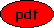Singularities of Algebraic Differential Equations Co-author(s): Markus Lange-Hegermann and Daniel Robertz and Matthias Seiß Reference: Advances in Applied Mathematics, 131 (2021) 102266 Description: This paper combines algebraic and geometric approaches to general systems of algebraic ordinary or partial differential equations to provide a unified framework for the definition and detection of singularities of a given system at a fixed order. The three main results are firstly a proof that even in the case of partial differential equations regular points are generic. Secondly, an algorithm for the effective detection of all singularities at a given order or, more precisely, for the determination of a regularity decomposition is presented. Finally, a rigorous definition of a regular differential equation, a notion that is ubiquitous in the geometric theory of differential equations, is given and it is shown that the above mentioned algorithm extracts from each prime component a regular differential equation. Our main algorithmic tools are on the one hand the algebraic resp. differential Thomas decomposition and on the other hand the Vessiot theory of differential equations. PDF File:(1205 kB) Home, Last update: Mon Aug 30 07:55:56 2021